Download Presentation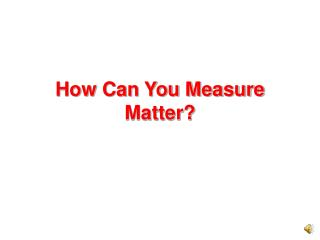How Can You Measure Matter?

# How Can You Measure Matter? - PowerPoint PPT Presentation

How Can You Measure Matter?. To measure matter, systems of standard units have been developed. A standard unit is a unit of measure that people agree to use, such as the inch, pound, yard, and gallon. These are units in the English system, which is mostly used in the United States.I am the owner, or an agent authorized to act on behalf of the owner, of the copyrighted work described.
Download Presentation## How Can You Measure Matter?

An Image/Link below is provided (as is) to download presentation

Download Policy: Content on the Website is provided to you AS IS for your information and personal use and may not be sold / licensed / shared on other websites without getting consent from its author.While downloading, if for some reason you are not able to download a presentation, the publisher may have deleted the file from their server.

- - - - - - - - - - - - - - - - - - - - - - - - - - E N D - - - - - - - - - - - - - - - - - - - - - - - - - -
Presentation Transcript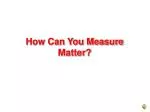### How Can You Measure Matter?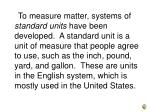To measure matter, systems of standard units have been developed. A standard unit is a unit of measure that people agree to use, such as the inch, pound, yard, and gallon. These are units in the English system, which is mostly used in the United States.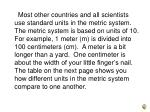Most other countries and all scientists use standard units in the metric system. The metric system is based on units of 10. For example, 1 meter (m) is divided into 100 centimeters (cm). A meter is a bit longer than a yard. One centimeter is about the width of your little finger’s nail. The table on the next page shows you how different units in the metric system compare to one another.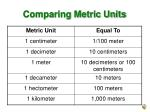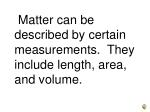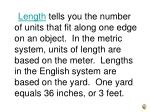Length tells you the number of units that fit along one edge on an object. In the metric system, units of length are based on the meter. Lengths in the English system are based on the yard. One yard equals 36 inches, or 3 feet.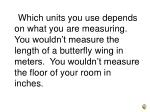Which units you use depends on what you are measuring. You wouldn’t measure the length of a butterfly wing in meters. You wouldn’t measure the floor of your room in inches.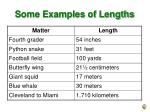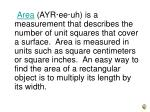Area (AYR·ee·uh) is a measurement that describes the number of unit squares that cover a surface. Area is measured in units such as square centimeters or square inches. An easy way to find the area of a rectangular object is to multiply its length by its width.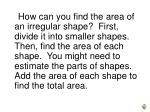How can you find the area of an irregular shape? First, divide it into smaller shapes. Then, find the area of each shape. You might need to estimate the parts of shapes. Add the area of each shape to find the total area.Volume (VAHL·yewm) describes how much space an object takes up. It measures the number of cubes that can fit in an object. Solids are measured in units such as cubic centimeters or cubic inches. To find the volume of a solid rectangle, multiply its length by its width and its height.You can also measure the volume of a liquid. In the metric system, it is measured in units called liters (L). One liter is made up of 1,000 milliliters (mL). One mL of a liquid takes up the same amount of space as a 1-cm cube.You can use water to measure the volume of a solid. First, measure some water. Then, completely submerge the object. Subtract the original water level from the new water level. The water level rises by the exact volume of the object.• How can you use a graduated cylinder and water to measure the volume of a rock?What are three measurements of matter you can make?
• Answer the question…
• With a partner
• In your Learning NotebookUsing Graphic Sources
• How many centimeters are in one meter?
• How many meters are in one decameter?
• How many meters would it take to equal one hectometer?
• How long is a giant squid?
• Which is longer – a python snake or a football field?
• By how many feet?length
• The number of units that fit along one edge of an object.

Back to textarea
• The number of unit squares that fit inside a surface.

Back to textvolume
• The amount of space an object takes up.

Back to text